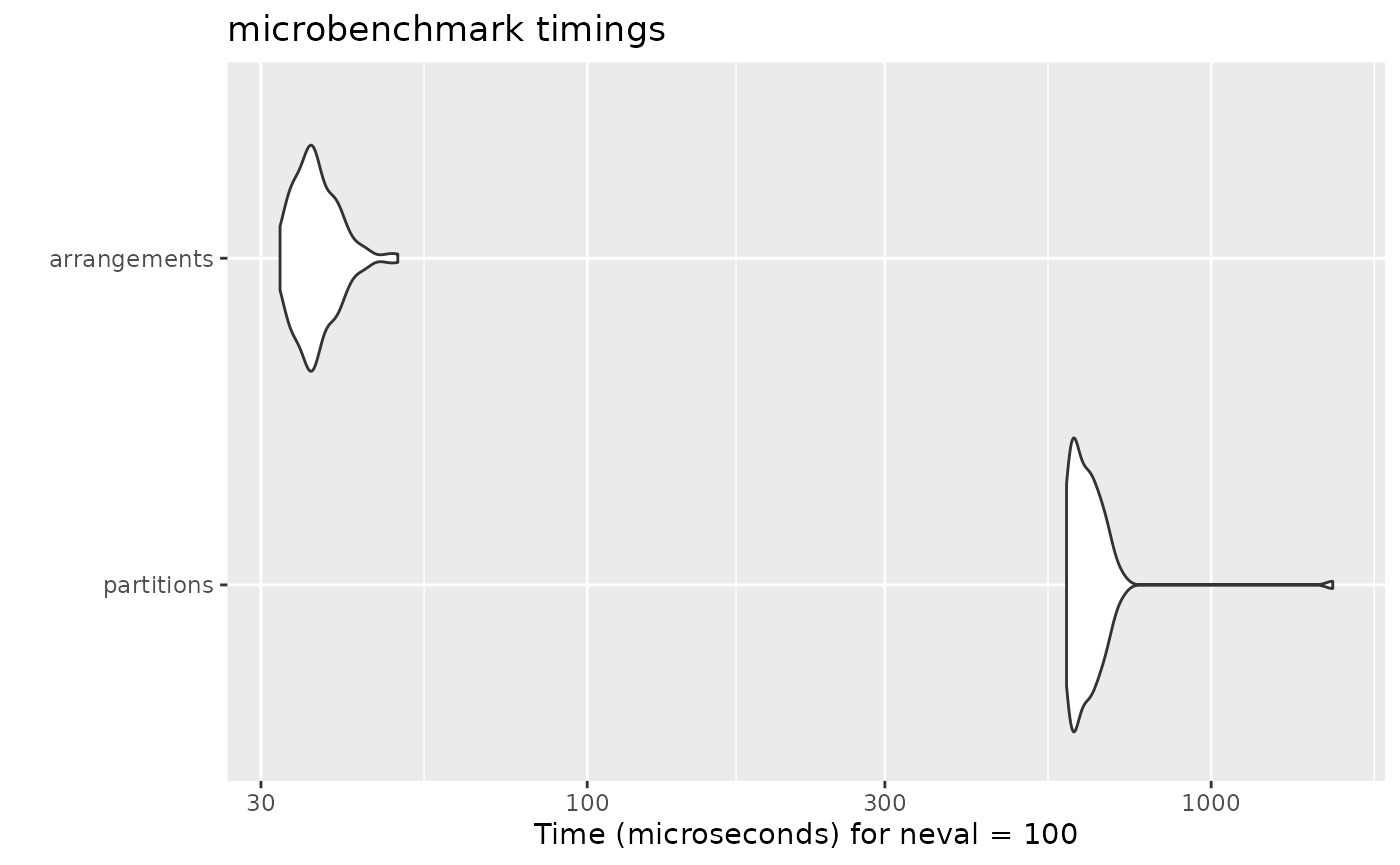The goal of this document is to compare the performances of some of the existing packages for generating combinations, permutations and partitions.

library(gtools)
library(combinat)
library(multicool)
library(partitions)
library(permute)
library(permutations)
library(RcppAlgos)
library(arrangements)
gtools.permutations <- gtools::permutations
gtools.combinations <- gtools::combinations
partitions.compositions <- partitions::compositions
utils.combn <- utils::combn

gtools::permutations, gtools::combinations, partitions::compositions and utils::combn are renamed because they are being overwritten by other packages.

### Permutations

Permutations of 6 items

autoplot(microbenchmark(
gtools = gtools.permutations(6, 6),
multicool = allPerm(initMC(1:6)),
partitions = partitions::perms(6),
permuate = allPerms(6),
permutations = allperms(6),
RcppAlgos = permuteGeneral(6, 6),
arrangements = permutations(6)
))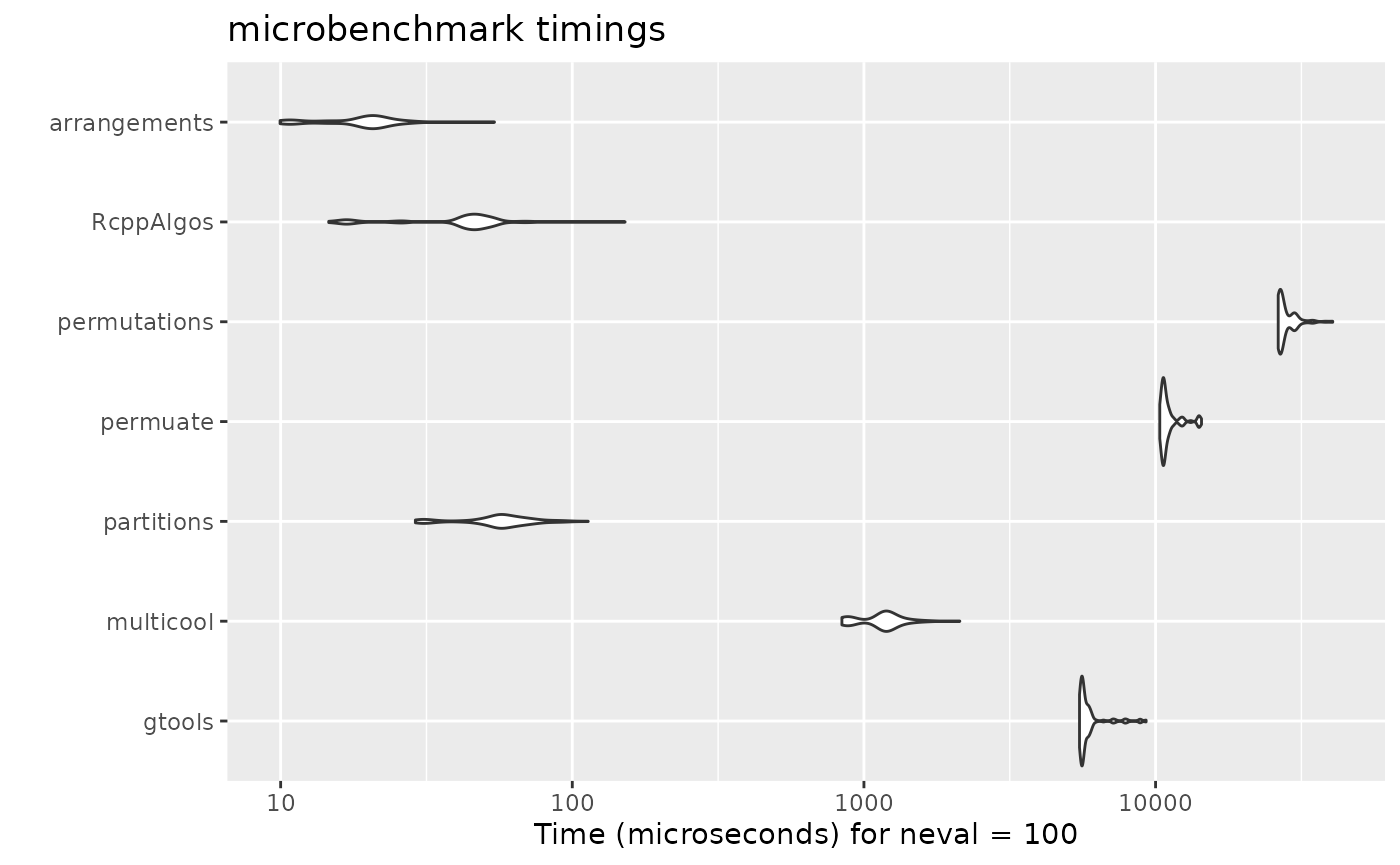5-Permutations of 7 items

autoplot(microbenchmark(
gtools = gtools.permutations(7, 5),
RcppAlgos = permuteGeneral(7, 5),
arrangements = permutations(7, 5)
))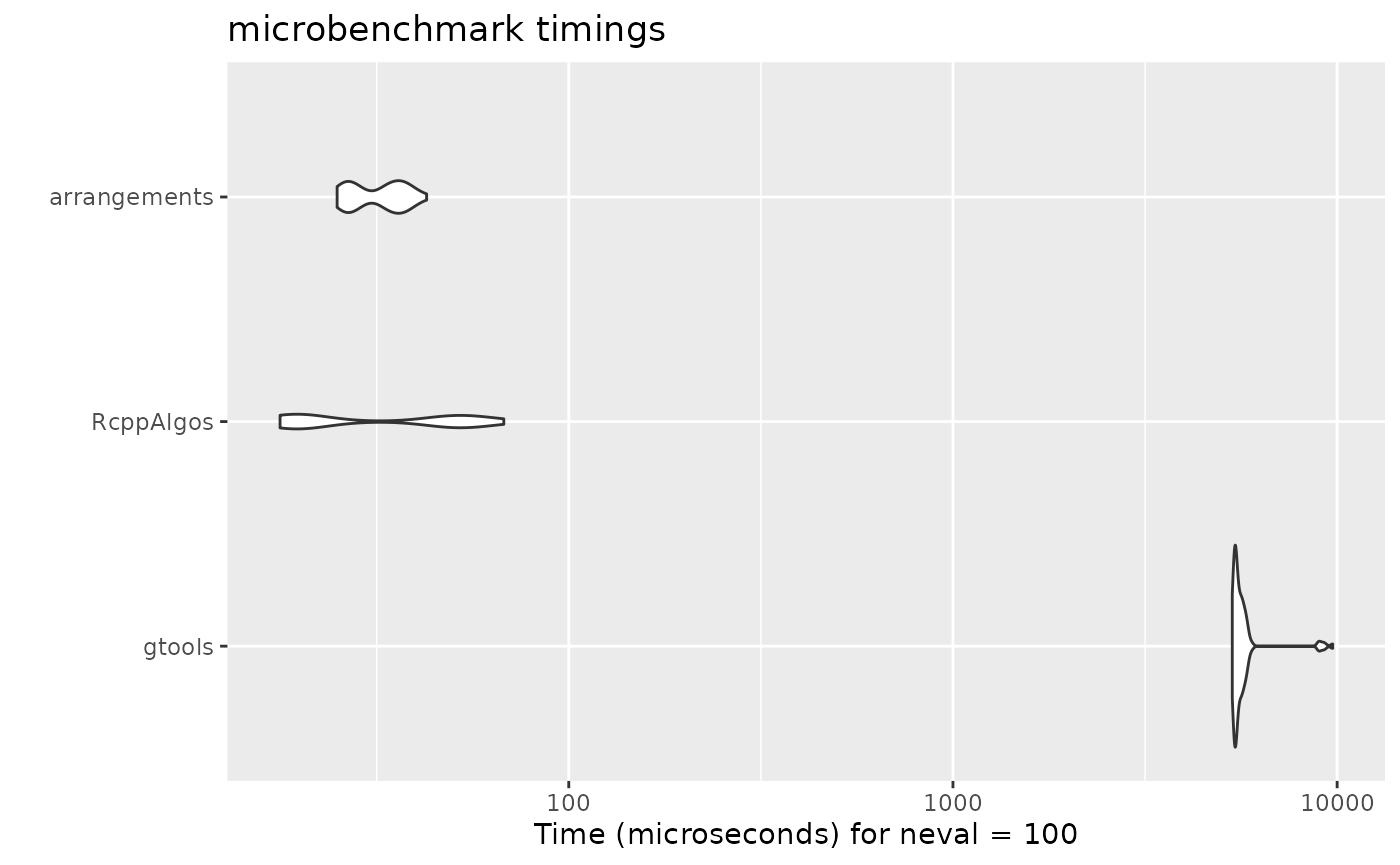Permutations of multiset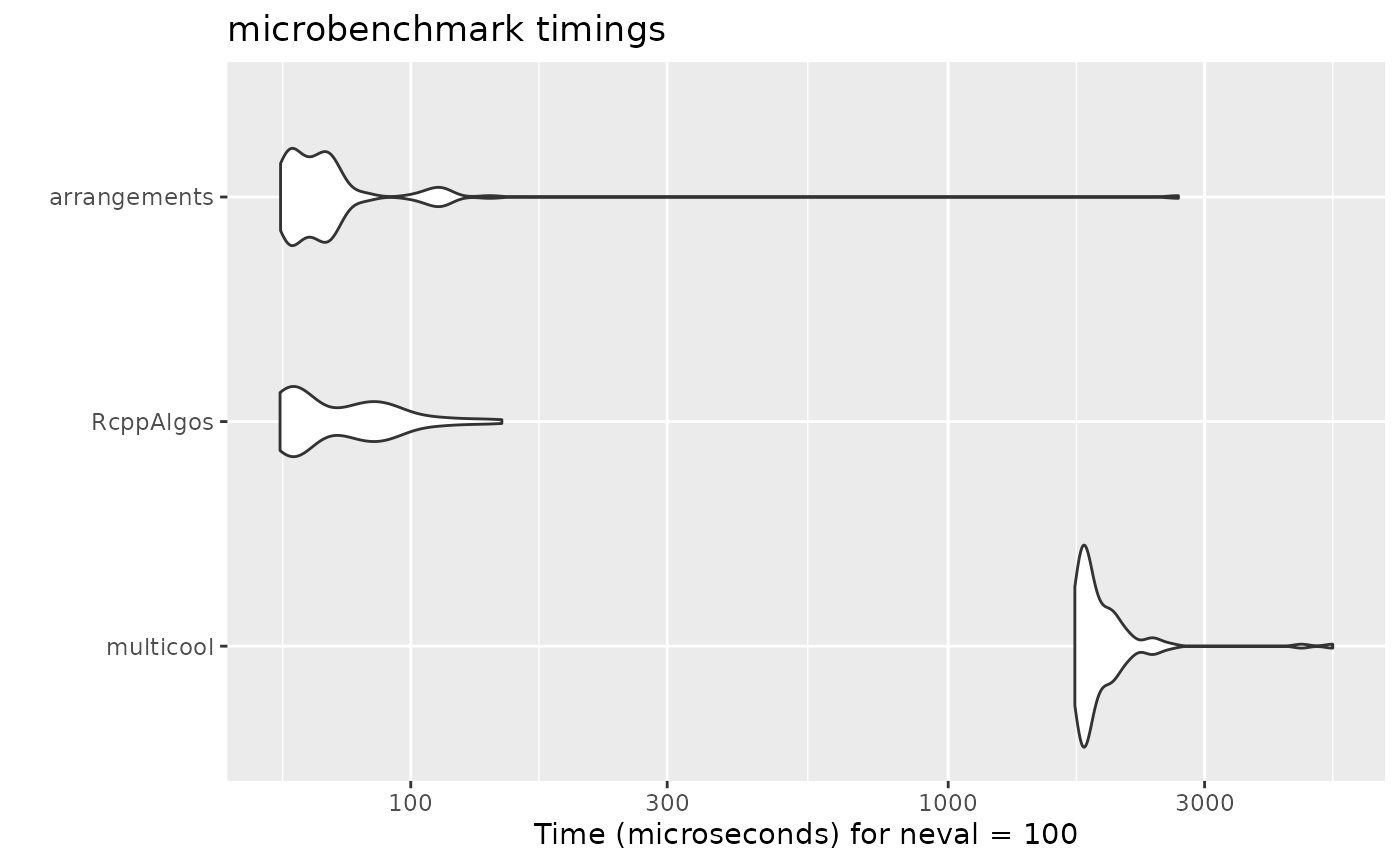K-Permutations of multiset

f <- c(2, 1, 2, 1, 2)
x <- rep(1:5, f)
autoplot(microbenchmark(
multicool = allPerm(initMC(x)),
RcppAlgos = permuteGeneral(5, 6, freqs = f),
arrangements = permutations(k = 6, freq = f)
))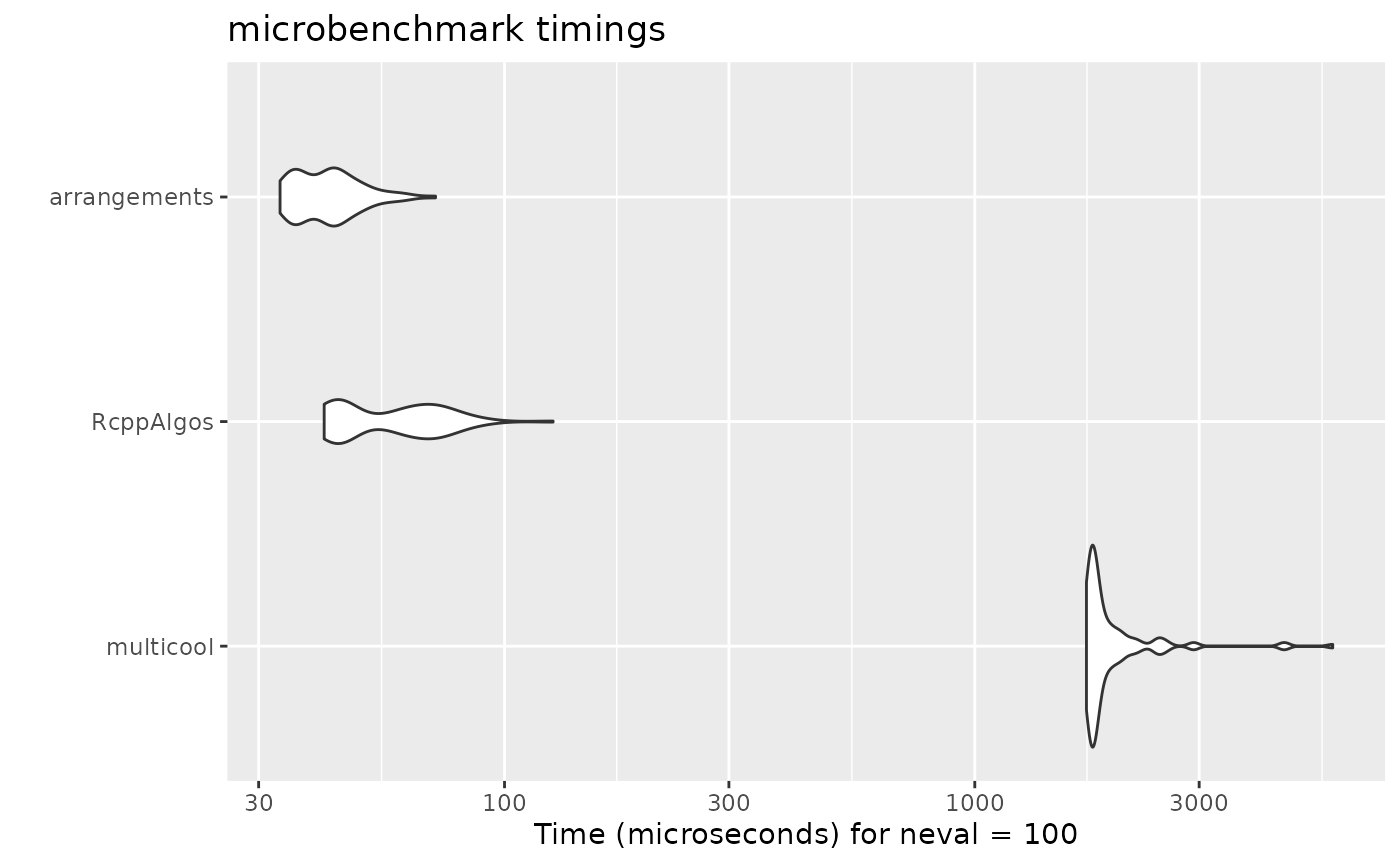### Combinations

7-Combinations of 15 items

autoplot(microbenchmark(
gtools = gtools.combinations(15, 7),
combinat = combn(15, 7),
utils = utils.combn(15, 7),
RcppAlgos = comboGeneral(15, 7),
arrangements = combinations(15, 7)
))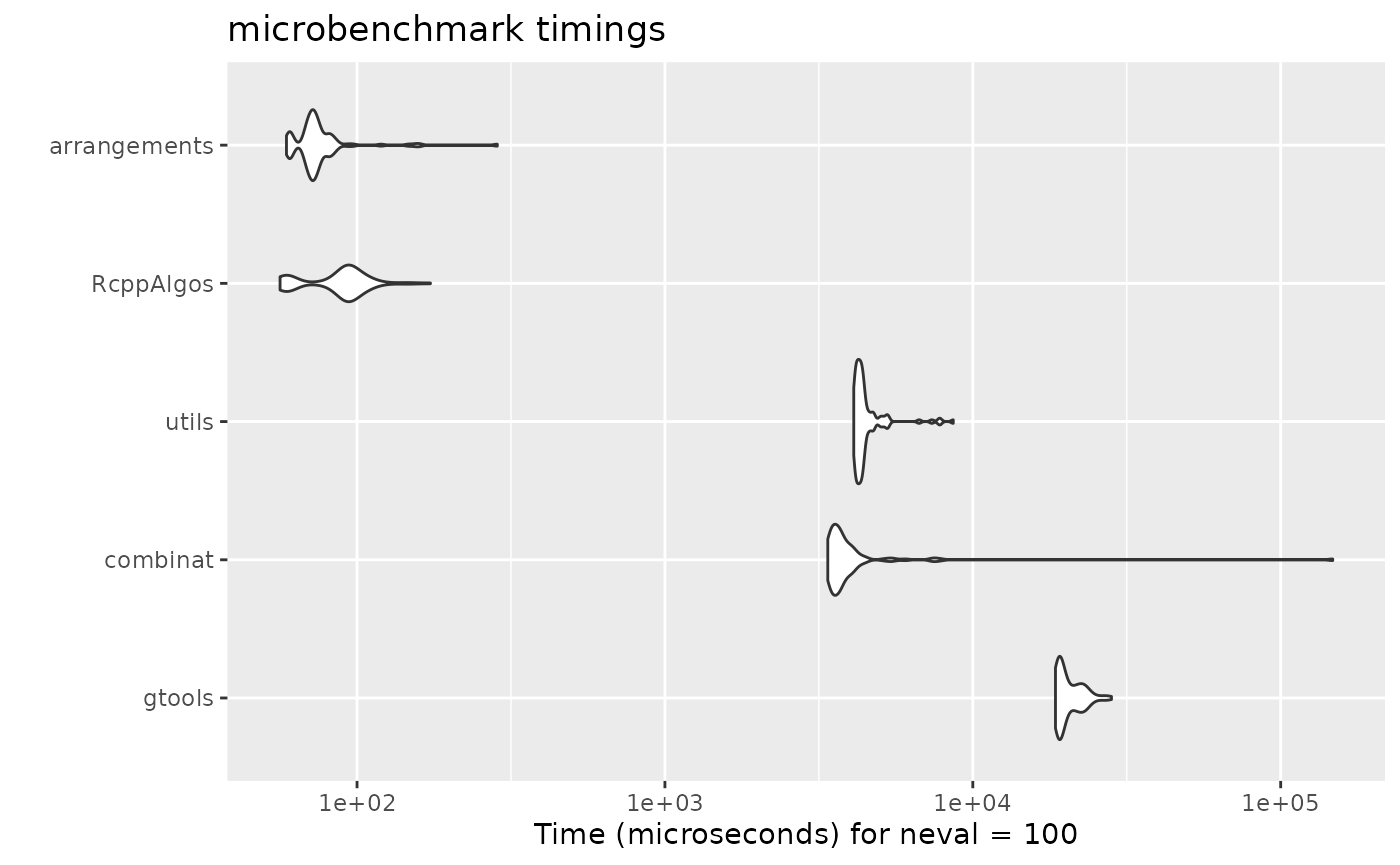Combinations with replacement of 9 items

autoplot(microbenchmark(
gtools = gtools.combinations(9, 6, repeats.allowed = TRUE),
RcppAlgos = comboGeneral(9, 6, repetition = TRUE),
arrangements = combinations(9, 6, replace = TRUE)
))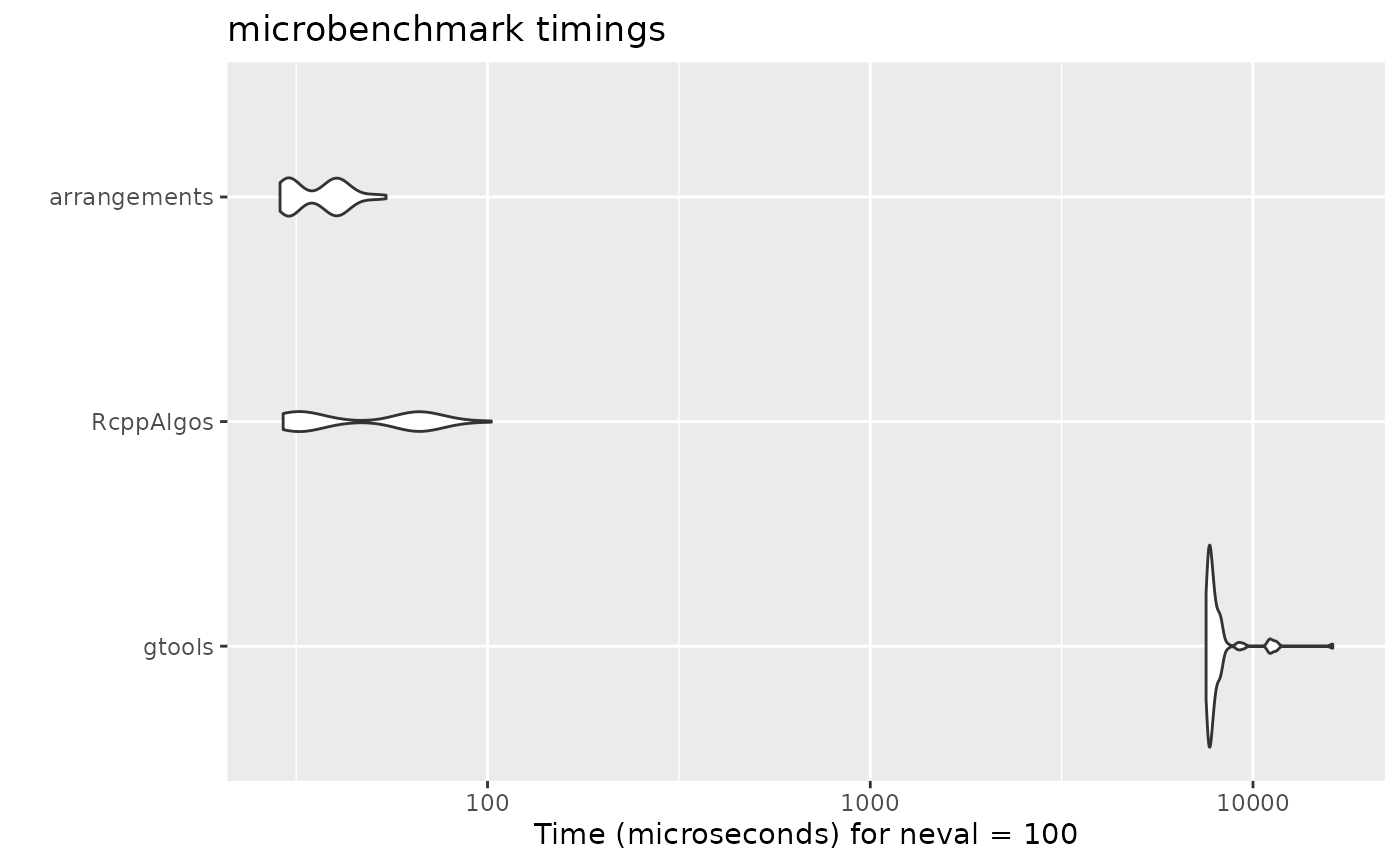Combinations of multiset

f <- c(2, 1, 2, 1, 2, 2, 1, 2)
x <- rep(1:8, f)
autoplot(microbenchmark(
RcppAlgos = comboGeneral(8, 6, freqs = f),
arrangements = combinations(k = 6, freq = f)
))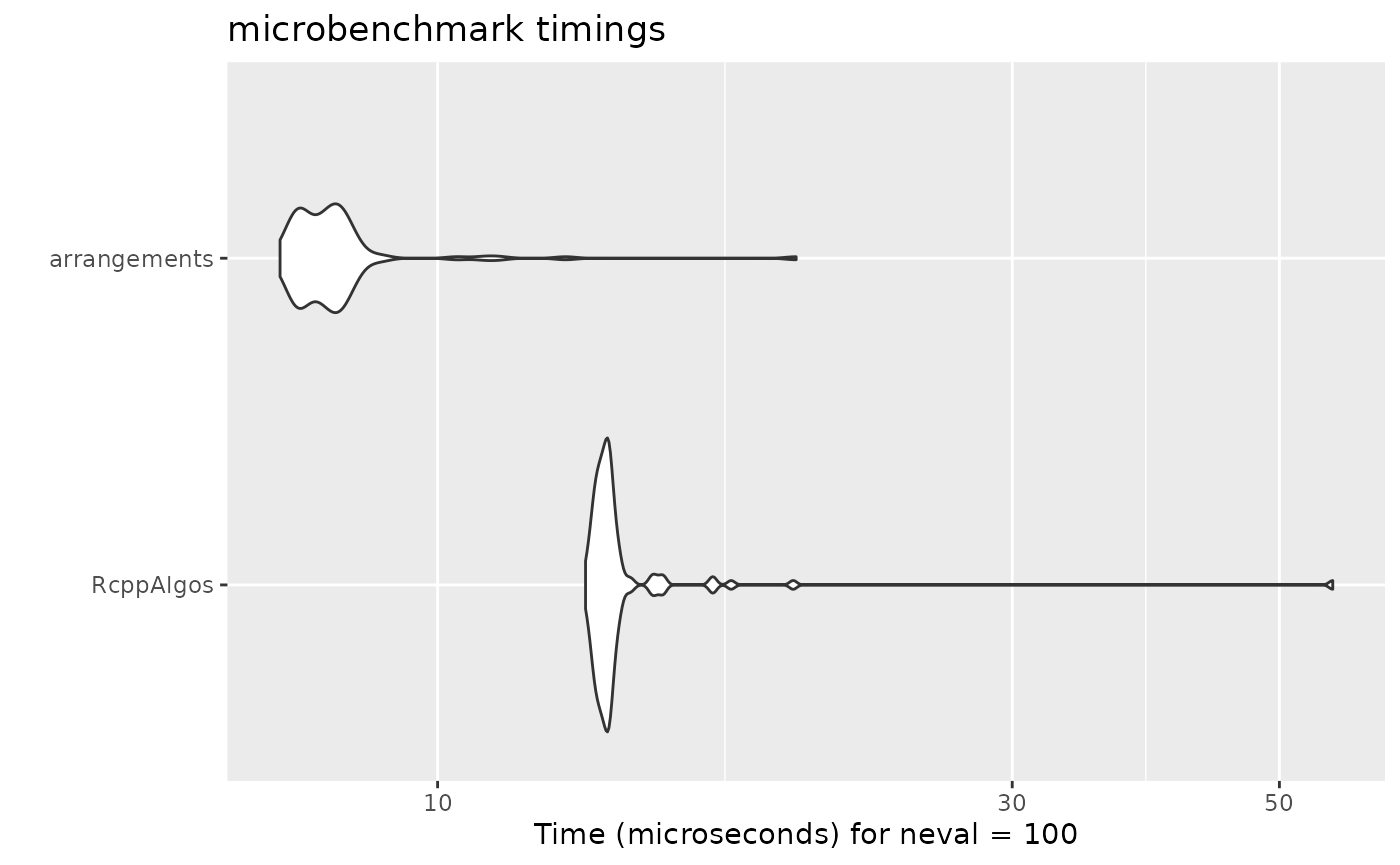### Partitions

Partitions of 30

autoplot(microbenchmark(
partitions = parts(30),
arrangements = partitions(30)
))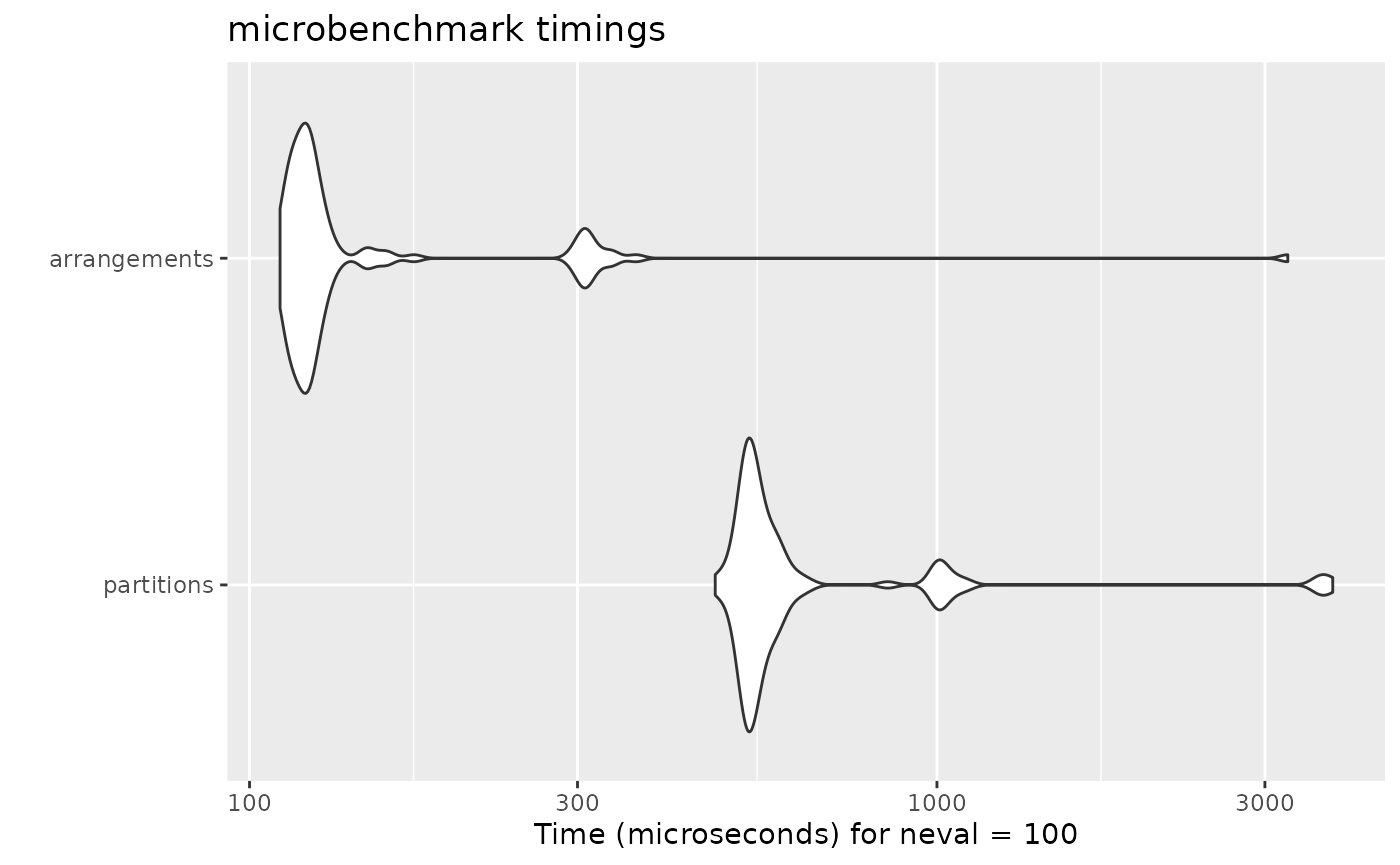Partitions of 50 into 10 parts

autoplot(microbenchmark(
partitions = restrictedparts(50, 10, include.zero = FALSE),
arrangements = partitions(50, 10)
))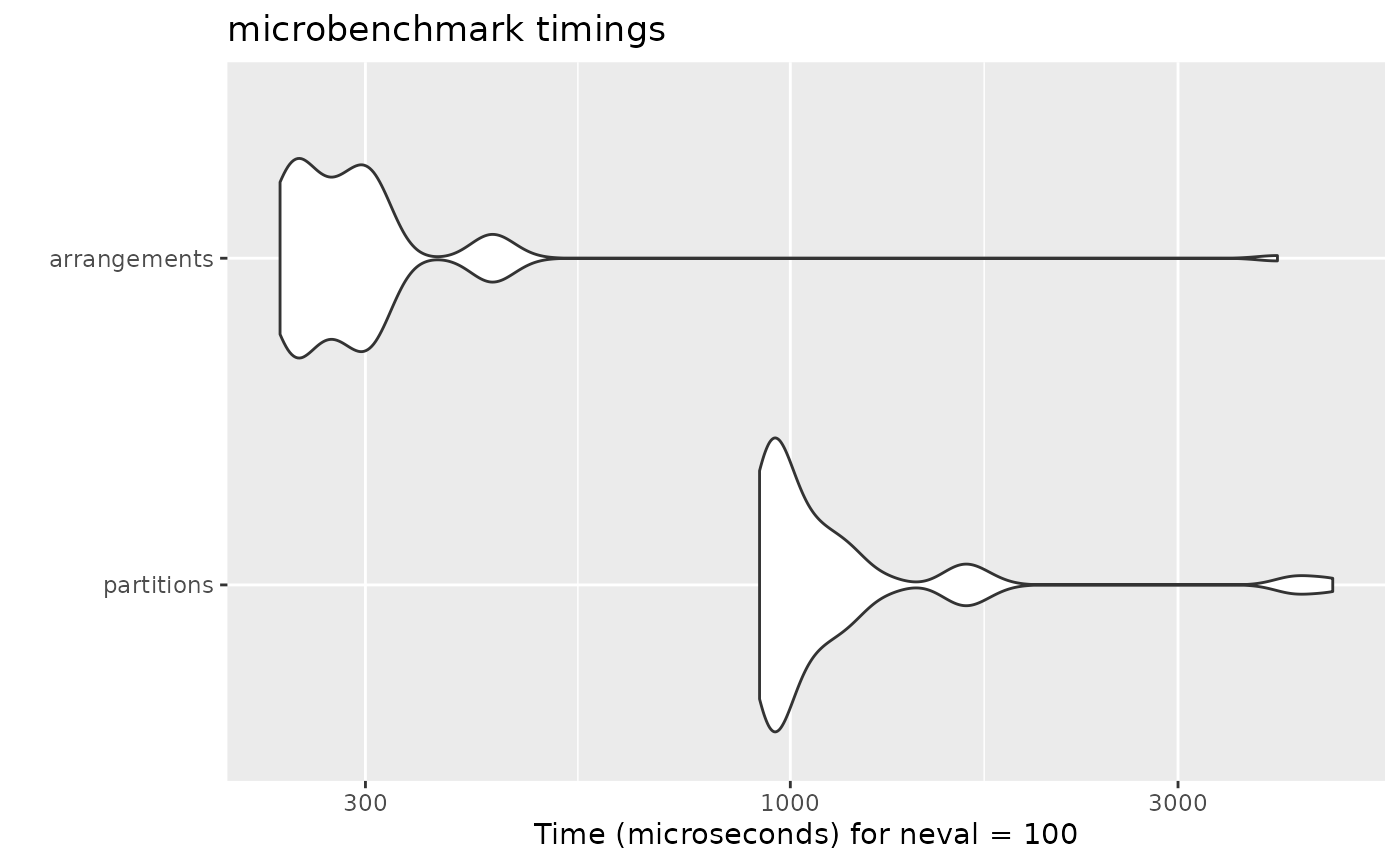Partitions of 50 into distinct parts

autoplot(microbenchmark(
partitions = diffparts(50),
arrangements = partitions(50, distinct = TRUE)
))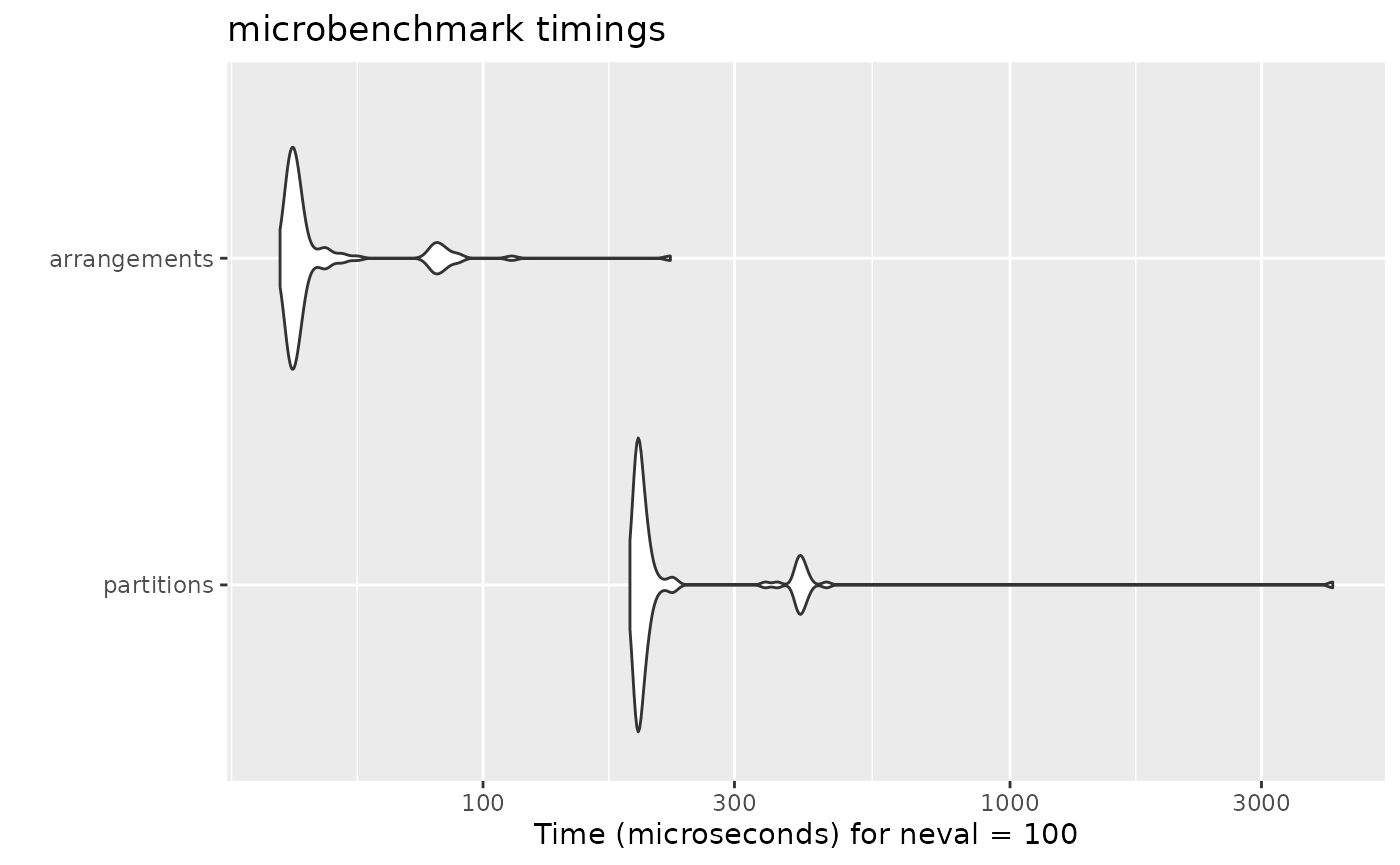### Compositions

Compositions of 8

autoplot(microbenchmark(
partitions = partitions.compositions(8, include.zero = FALSE),
arrangements = compositions(8)
))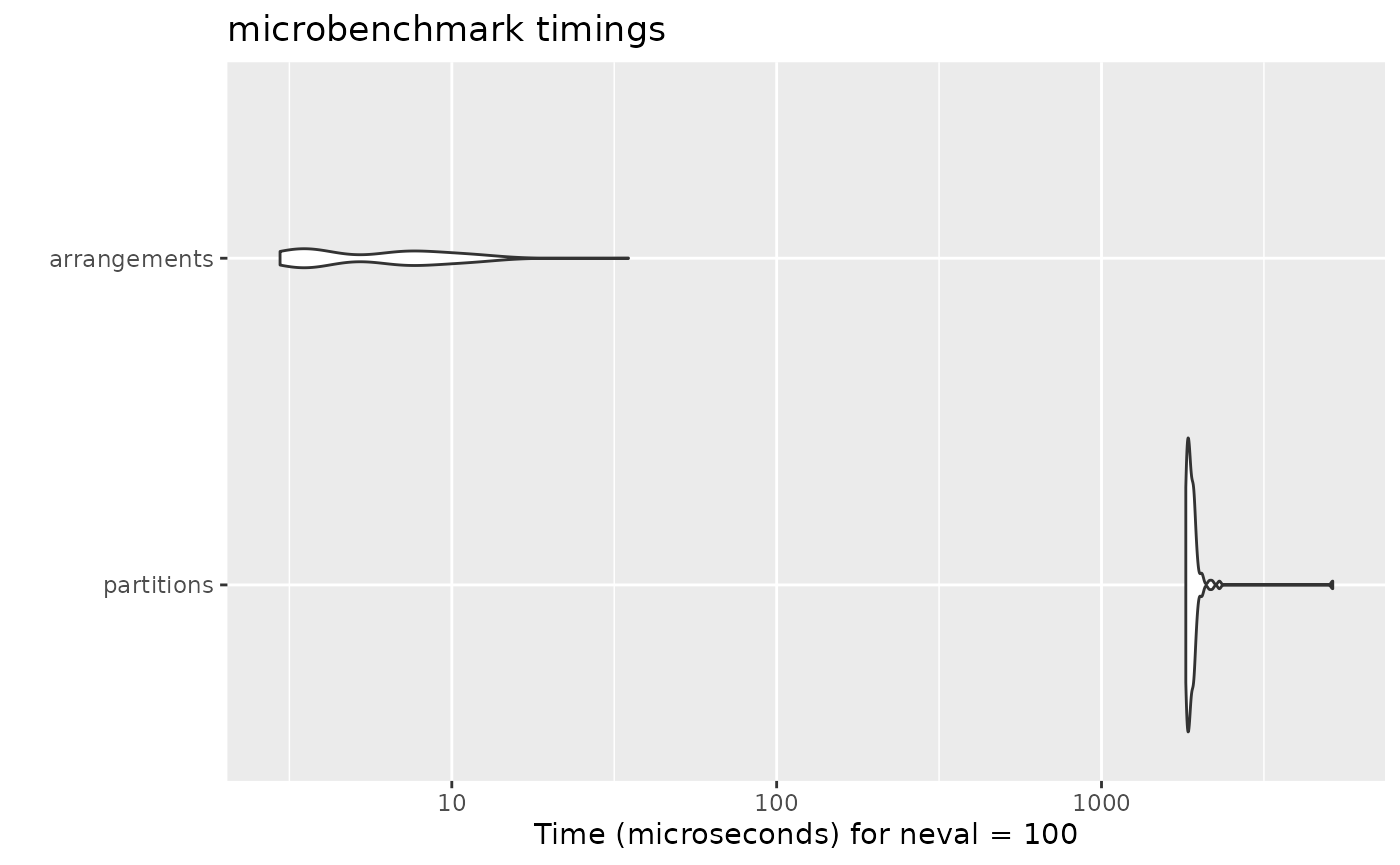Compositions of 20 into 5 parts

autoplot(microbenchmark(
partitions = partitions.compositions(20, 5, include.zero = FALSE),
arrangements = compositions(20, 5)
))